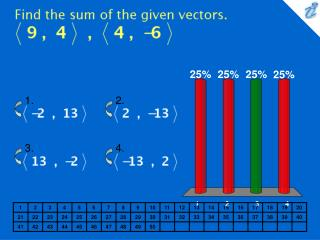DownloadDownload PresentationFind the sum of the given vectors. {image}

# Find the sum of the given vectors. {image}

Download Presentation## Find the sum of the given vectors. {image}

- - - - - - - - - - - - - - - - - - - - - - - - - - - E N D - - - - - - - - - - - - - - - - - - - - - - - - - - -
##### Presentation Transcript

1. 1. 2. 3. 4. Find the sum of the given vectors. {image} • {image} • {image} • {image} • {image}

2. Find 7a + 4b . a = 7i - 6j , b = 6i + 3j • 73i - 30j • 75i + 30j • 73i + 30j • 74i - 30j • 74i - 29j

3. 1. 2. 3. 4. Find a unit vector with the same direction as 3i - 5j + 2k. • {image} • 3i - j + k • {image} • i - j + k

4. A woman walks due west on the deck of a ship at 2 mi/h. The ship is moving north at a speed of 25 mi/h. Find the speed of the woman relative to the surface of the water. Round the result to the nearest tenth. • 26.2 mi/h • 25 mi/h • 27.1 mi/h • 23.1 mi/h • 25.1 mi/h